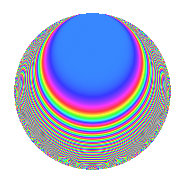# Properties

 Label 1800.1.crLevel 1800 Weight 1 Character orbit cr Rep. character $$\chi_{1800}(37,\cdot)$$ Character field $$\Q(\zeta_{20})$$ Dimension 16 Newforms 1 Sturm bound 360 Trace bound 0

# Related objects

## Defining parameters

 Level: $$N$$ = $$1800 = 2^{3} \cdot 3^{2} \cdot 5^{2}$$ Weight: $$k$$ = $$1$$ Character orbit: $$[\chi]$$ = 1800.cr (of order $$20$$ and degree $$8$$) Character conductor: $$\operatorname{cond}(\chi)$$ = $$200$$ Character field: $$\Q(\zeta_{20})$$ Newforms: $$1$$ Sturm bound: $$360$$ Trace bound: $$0$$

## Dimensions

The following table gives the dimensions of various subspaces of $$M_{1}(1800, [\chi])$$.

Total New Old
Modular forms 80 32 48
Cusp forms 16 16 0
Eisenstein series 64 16 48

The following table gives the dimensions of subspaces with specified projective image type.

$$D_n$$ $$A_4$$ $$S_4$$ $$A_5$$
Dimension 16 0 0 0

## Trace form

 $$16q$$ $$\mathstrut +\mathstrut 4q^{7}$$ $$\mathstrut +\mathstrut O(q^{10})$$ $$16q$$ $$\mathstrut +\mathstrut 4q^{7}$$ $$\mathstrut -\mathstrut 4q^{10}$$ $$\mathstrut +\mathstrut 4q^{16}$$ $$\mathstrut -\mathstrut 4q^{22}$$ $$\mathstrut -\mathstrut 16q^{28}$$ $$\mathstrut +\mathstrut 4q^{55}$$ $$\mathstrut +\mathstrut 4q^{58}$$ $$\mathstrut +\mathstrut 4q^{70}$$ $$\mathstrut -\mathstrut 4q^{73}$$ $$\mathstrut -\mathstrut 4q^{88}$$ $$\mathstrut -\mathstrut 16q^{97}$$ $$\mathstrut +\mathstrut O(q^{100})$$

## Decomposition of $$S_{1}^{\mathrm{new}}(1800, [\chi])$$ into irreducible Hecke orbits

Label Dim. $$A$$ Field Image CM RM Traces $q$-expansion
$$a_2$$ $$a_3$$ $$a_5$$ $$a_7$$
1800.1.cr.a $$16$$ $$0.898$$ $$\Q(\zeta_{40})$$ $$D_{20}$$ $$\Q(\sqrt{-6})$$ None $$0$$ $$0$$ $$0$$ $$4$$ $$q-\zeta_{40}^{19}q^{2}-\zeta_{40}^{18}q^{4}+\zeta_{40}^{9}q^{5}+\cdots$$